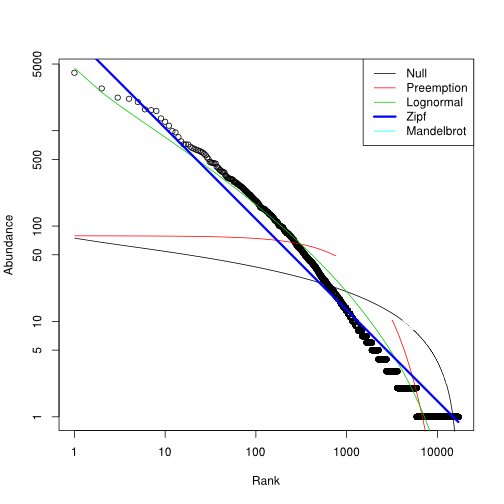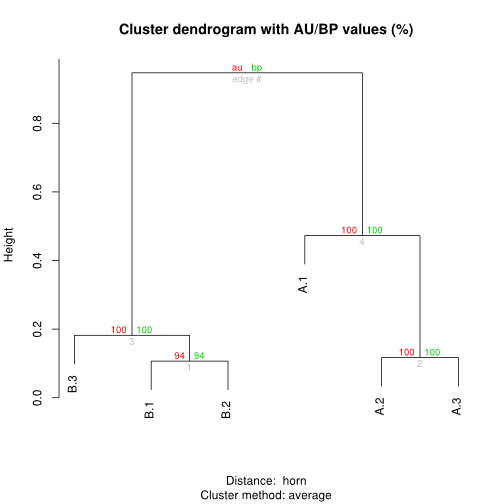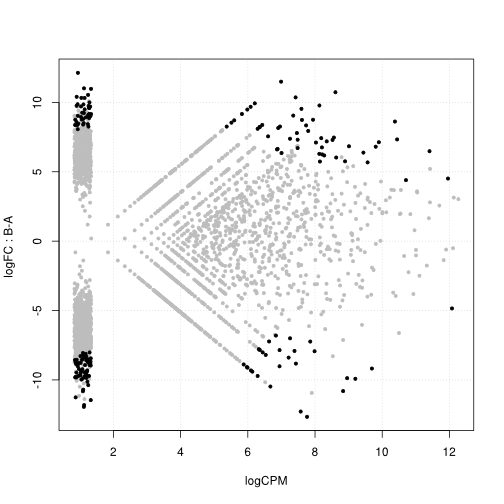# Gallery

### Histogram of alignment scores

Assuming a single library loaded in a data frame called clonotypes with the `read clonotypes()` function:

```hist(log(clonotypes\$score)
```### Scale-free distribution of the clonotype expression levels

Assuming table a of clonotype counts produced with the `clonotype table()` function, the following commands study the first library in that table.

```library(vegan)
```### Grouping libraries by bootstraped hierarchical clustering

Assuming table a of clonotype counts produced with the `clonotype table()` function, containing six libraries (for instance two biological triplicates).```library(pvclust)
plot(pvclust(a, n=1000))
```

### Differential expression analysis

Assuming table a of clonotype counts produced with the `clonotype table()` function, containing six libraries (for instance two biological triplicates).

This representation is similar to MA plots used in microarray and digital expression analysis, where the x axis represents the average expression strength, and the y axis represents the fold change (both on a logarithmic scale). Black dots represent clonotypes over-represented in one population compared to the other.

```library(edgeR)
a.dge <- DGEList(counts=a,group=c("B","A","A","B","A","B"))
a.dge <- estimateTagwiseDisp(DGEList(counts=a,group=c("B","A","A","B","A","B")))
a.dge.com <- exactTest(a.dge)
detags <- function (COMPARISON, ADJUST="fdr", P=0.05, SORTBY="PValue", DIRECTION="all") {
if (DIRECTION == "all") {
}
if (DIRECTION == "up") {